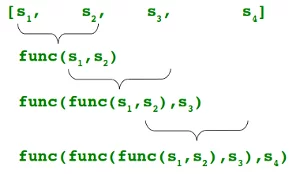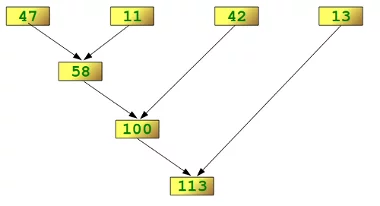## Lambda, filter, reduce and mapIf Guido van Rossum, the author of the programming language Python, had got his will, this chapter would have been missing in our tutorial. In his article from May 2005 "All Things Pythonic: The fate of reduce() in Python 3000", he gives his reasons for dropping lambda, map(), filter() and reduce(). He expected resistance from the Lisp and the scheme "folks". What he didn't anticipate was the rigidity of this opposition. Enough that Guido van Rossum wrote hardly a year later: "After so many attempts to come up with an alternative for lambda, perhaps we should admit defeat. I've not had the time to follow the most recent rounds, but I propose that we keep lambda, so as to stop wasting everybody's talent and time on an impossible quest." We can see the result: lambda, map() and filter() are still part of core Python. Only reduce() had to go; it moved into the module functools.

His reasoning for dropping them is like this:

• There is an equally powerful alternative to lambda, filter, map and reduce, i.e. list comprehension
• List comprehension( is more evident and easier to understand
• Having both list comprehension and "Filter, map, reduce and lambda" is transgressing the Python motto "There should be one obvious way to solve a problem"

Some like it, others hate it and many are afraid of the lambda operator. The lambda operator or lambda function is a way to create small anonymous functions, i.e. functions without a name. These functions are throw-away functions, i.e. they are just needed where they have been created. Lambda functions are mainly used in combination with the functions filter(), map() and reduce(). The lambda feature was added to Python due to the demand from Lisp programmers.

The general syntax of a lambda function is quite simple:

lambda argument_list: expression

The argument list consists of a comma separated list of arguments and the expression is an arithmetic expression using these arguments. You can assign the function to a variable to give it a name.

The following example of a lambda function returns the sum of its two arguments:

sum = lambda x, y : x + y
sum(3,4)

Output:
7

The above example might look like a plaything for a mathematician. A formalism which turns an easy to comprehend issue into an abstract harder to grasp formalism. Above all, we could have had the same effect by just using the following conventional function definition:

def sum(x,y):
return x + y

sum(3,4)

Output:
7

We can assure you that the advantages of this approach will be apparent, when you will have learnt to use the map() function.

### The map() Function

As we have mentioned earlier, the advantage of the lambda operator can be seen when it is used in combination with the map() function. map() is a function which takes two arguments:

r = map(func, seq)

The first argument func is the name of a function and the second a sequence (e.g. a list) seq. map() applies the function func to all the elements of the sequence seq. Before Python3, map() used to return a list, where each element of the result list was the result of the function func applied on the corresponding element of the list or tuple "seq". With Python 3, map() returns an iterator.

The following example illustrates the way of working of map():

def fahrenheit(T):
return ((float(9)/5)*T + 32)

def celsius(T):
return (float(5)/9)*(T-32)

temperatures = (36.5, 37, 37.5, 38, 39)
F = map(fahrenheit, temperatures)
C = map(celsius, F)
temperatures_in_Fahrenheit = list(map(fahrenheit, temperatures))
temperatures_in_Celsius = list(map(celsius, temperatures_in_Fahrenheit))
print(temperatures_in_Fahrenheit)
print(temperatures_in_Celsius)

[97.7, 98.60000000000001, 99.5, 100.4, 102.2]
[36.5, 37.00000000000001, 37.5, 38.00000000000001, 39.0]


In the example above we haven't used lambda. By using lambda, we wouldn't have had to define and name the functions fahrenheit() and celsius(). You can see this in the following interactive session:

C = [39.2, 36.5, 37.3, 38, 37.8]
F = list(map(lambda x: (float(9)/5)*x + 32, C))
print(F)

[102.56, 97.7, 99.14, 100.4, 100.03999999999999]

C = list(map(lambda x: (float(5)/9)*(x-32), F))
print(C)

[39.2, 36.5, 37.300000000000004, 38.00000000000001, 37.8]


map() can be applied to more than one list. The lists don't have to have the same length. map() will apply its lambda function to the elements of the argument lists, i.e. it first applies to the elements with the 0th index, then to the elements with the 1st index until the n-th index is reached:

a = [1, 2, 3, 4]
b = [17, 12, 11, 10]
c = [-1, -4, 5, 9]

list(map(lambda x, y, z : x+y+z, a, b, c))

Output:
[17, 10, 19, 23]
list(map(lambda x, y : x+y, a, b))

Output:
[18, 14, 14, 14]
list(map(lambda x, y, z : 2.5*x + 2*y - z, a, b, c))

Output:
[37.5, 33.0, 24.5, 21.0]

If one list has fewer elements than the others, map will stop when the shortest list has been consumed:

a = [1, 2, 3]
b = [17, 12, 11, 10]
c = [-1, -4, 5, 9]

list(map(lambda x, y, z : 2.5*x + 2*y - z, a, b, c))

Output:
[37.5, 33.0, 24.5]

We can see in the example above that the parameter x gets its values from the list a, while y gets its values from b, and z from list c.

### Mapping a List of Functions

The map function of the previous chapter was used to apply one function to one or more iterables. We will now write a function which applies a bunch of functions, which may be an iterable such as a list or a tuple, for example, to one Python object.

from math import sin, cos, tan, pi

def map_functions(x, functions):
""" map an iterable of functions on the the object x """
res = []
for func in functions:
res.append(func(x))
return res

family_of_functions = (sin, cos, tan)
print(map_functions(pi, family_of_functions))

[1.2246467991473532e-16, -1.0, -1.2246467991473532e-16]


The previously defined map_functions function can be simplified with the list comprehension technique, which we will cover in the chapter list comprehension:

def map_functions(x, functions):
return [ func(x) for func in functions ] 

### Filtering

The function

filter(function, sequence)

offers an elegant way to filter out all the elements of a sequence "sequence", for which the function function returns True. i.e. an item will be produced by the iterator result of filter(function, sequence) if item is included in the sequence "sequence" and if function(item) returns True.

In other words: The function filter(f,l) needs a function f as its first argument. f has to return a Boolean value, i.e. either True or False. This function will be applied to every element of the list l. Only if f returns True will the element be produced by the iterator, which is the return value of filter(function, sequence).

In the following example, we filter out first the odd and then the even elements of the sequence of the first 11 Fibonacci numbers:

fibonacci = [0,1,1,2,3,5,8,13,21,34,55]
odd_numbers = list(filter(lambda x: x % 2, fibonacci))
print(odd_numbers)

[1, 1, 3, 5, 13, 21, 55]

even_numbers = list(filter(lambda x: x % 2 == 0, fibonacci))
print(even_numbers)

[0, 2, 8, 34]

even_numbers = list(filter(lambda x: x % 2 -1, fibonacci))
print(even_numbers)

[0, 2, 8, 34]


### Reducing a List

As we mentioned in the introduction of this chapter of our tutorial. reduce() had been dropped from the core of Python when migrating to Python 3. Guido van Rossum hates reduce(), as we can learn from his statement in a posting, March 10, 2005, in artima.com:

"So now reduce(). This is actually the one I've always hated most, because, apart from a few examples involving + or *, almost every time I see a reduce() call with a non-trivial function argument, I need to grab pen and paper to diagram what's actually being fed into that function before I understand what the reduce() is supposed to do. So in my mind, the applicability of reduce() is pretty much limited to associative operators, and in all other cases it's better to write out the accumulation loop explicitly."



The function

reduce(func, seq)

continually applies the function func() to the sequence seq. It returns a single value.

If seq = [ s1, s2, s3, ... , sn ], calling reduce(func, seq) works like this:

At first the first two elements of seq will be applied to func, i.e. func(s1,s2) The list on which reduce() works looks now like this: [ func(s1, s2), s3, ... , sn ]
In the next step func will be applied on the previous result and the third element of the list, i.e. func(func(s1, s2),s3)
The list looks like this now: [ func(func(s1, s2),s3), ... , sn ]
Continues like this until just one element is left and returns this element as the result of reduce()



If n is equal to 4 the previous explanation can be illustrated like this:We want to illustrate this way of working of reduce() with a simple example. We have to import functools to be capable of using reduce:

import functools
functools.reduce(lambda x,y: x+y, [47,11,42,13])

Output:
113

The following diagram shows the intermediate steps of the calculation:### Examples of reduce()

Determining the maximum of a list of numerical values by using reduce:

from functools import reduce
f = lambda a,b: a if (a > b) else b
reduce(f, [47,11,42,102,13])

Output:
102

Calculating the sum of the numbers from 1 to 100:

from functools import reduce
reduce(lambda x, y: x+y, range(1,101))

Output:
5050

It's very simple to change the previous example to calculate the product (the factorial) from 1 to a number, but do not choose 100. We just have to turn the "+" operator into "*":

reduce(lambda x, y: x*y, range(1,49))

Output:
12413915592536072670862289047373375038521486354677760000000000

If you are into lottery, here are the chances to win a 6 out of 49 drawing:

reduce(lambda x, y: x*y, range(44,50))/reduce(lambda x, y: x*y, range(1,7))

Output:
13983816.0

### Exercises

1) Imagine an accounting routine used in a book shop. It works on a list with sublists, which look like this:

Order Number    Book Title and Author              Quantity     Price per Item
34587           Learning Python, Mark Lutz             4          40.95
98762           Programming Python, Mark Lutz          5          56.80
77226           Head First Python, Paul Barry          3          32.95
88112           Einführung in Python3, Bernd Klein     3          24.99

Write a Python program, which returns a list with 2-tuples. Each tuple consists of a the order number and the product of the price per items and the quantity. The product should be increased by 10,- € if the value of the order is smaller than 100,00 €.
Write a Python program using lambda and map.



2) The same bookshop, but this time we work on a different list. The sublists of our lists look like this: [ordernumber, (article number, quantity, price per unit), ... (article number, quantity, price per unit) ] Write a program which returns a list of two tuples with (order number, total amount of order).

### Solutions to the Exercises

orders = [ ["34587", "Learning Python, Mark Lutz", 4, 40.95],
["98762", "Programming Python, Mark Lutz", 5, 56.80],
["77226", "Head First Python, Paul Barry", 3,32.95],
["88112", "Einführung in Python3, Bernd Klein", 	3, 24.99]]

min_order = 100
invoice_totals = list(map(lambda x: x if x >= min_order else (x, x + 10),
map(lambda x: (x,x * x), orders)))

print(invoice_totals)

[('34587', 163.8), ('98762', 284.0), ('77226', 108.85000000000001), ('88112', 84.97)]

from functools import reduce

orders = [ [1, ("5464", 4, 9.99), ("8274",18,12.99), ("9744", 9, 44.95)],
[2, ("5464", 9, 9.99), ("9744", 9, 44.95)],
[3, ("5464", 9, 9.99), ("88112", 11, 24.99)],
[4, ("8732", 7, 11.99), ("7733",11,18.99), ("88112", 5, 39.95)] ]

min_order = 100
invoice_totals = list(map(lambda x: [x] + list(map(lambda y: y*y, x[1:])), orders))
invoice_totals = list(map(lambda x: [x] + [reduce(lambda a,b: a + b, x[1:])], invoice_totals))
invoice_totals = list(map(lambda x: x if x >= min_order else (x, x + 10), invoice_totals))

print (invoice_totals)

[[1, 678.3299999999999], [2, 494.46000000000004], [3, 364.79999999999995], [4, 492.57]]